Test: Capacitors & Inductors - 1

# Test: Capacitors & Inductors - 1

Test Description

## 15 Questions MCQ Test GATE Electrical Engineering (EE) 2023 Mock Test Series | Test: Capacitors & Inductors - 1

Test: Capacitors & Inductors - 1 for Electrical Engineering (EE) 2023 is part of GATE Electrical Engineering (EE) 2023 Mock Test Series preparation. The Test: Capacitors & Inductors - 1 questions and answers have been prepared according to the Electrical Engineering (EE) exam syllabus.The Test: Capacitors & Inductors - 1 MCQs are made for Electrical Engineering (EE) 2023 Exam. Find important definitions, questions, notes, meanings, examples, exercises, MCQs and online tests for Test: Capacitors & Inductors - 1 below.
Solutions of Test: Capacitors & Inductors - 1 questions in English are available as part of our GATE Electrical Engineering (EE) 2023 Mock Test Series for Electrical Engineering (EE) & Test: Capacitors & Inductors - 1 solutions in Hindi for GATE Electrical Engineering (EE) 2023 Mock Test Series course. Download more important topics, notes, lectures and mock test series for Electrical Engineering (EE) Exam by signing up for free. Attempt Test: Capacitors & Inductors - 1 | 15 questions in 30 minutes | Mock test for Electrical Engineering (EE) preparation | Free important questions MCQ to study GATE Electrical Engineering (EE) 2023 Mock Test Series for Electrical Engineering (EE) Exam | Download free PDF with solutions
 1 Crore+ students have signed up on EduRev. Have you?
Test: Capacitors & Inductors - 1 - Question 1

### An Inductor works as a ________ circuit for DC supply.

Detailed Solution for Test: Capacitors & Inductors - 1 - Question 1

Induced voltage across an inductor is zero if the current flowing through it is constant, i.e. Inductor works as a short circuit for DC supply.

Test: Capacitors & Inductors - 1 - Question 2

### A power factor of a circuit can be improved by placing which, among the following, in a circuit?

Detailed Solution for Test: Capacitors & Inductors - 1 - Question 2

Power factor = Real power / Apparent power = kW/kVA

Hence, the power factor is improved.

Test: Capacitors & Inductors - 1 - Question 3

### Which among the following equations is incorrect?

Detailed Solution for Test: Capacitors & Inductors - 1 - Question 3
• Q is directly proportional to V.
• The constant of proportionality in this case is C, that is, the capacitance.
• Hence Q=CV. From the given relation we can derive all the equations except for Q = C/V.
Test: Capacitors & Inductors - 1 - Question 4

What is the value of capacitance of a capacitor which has a voltage of 4V and has 16C of charge?

Detailed Solution for Test: Capacitors & Inductors - 1 - Question 4

Q is directly proportional to V.

The constant of proportionality in this case is C, that is, the capacitance.

Hence Q = CV

From the relation,

C = Q/V = 16/4 = 4F

Test: Capacitors & Inductors - 1 - Question 5

What is the total capacitance when two capacitors C1 and C2 are connected in series?

Detailed Solution for Test: Capacitors & Inductors - 1 - Question 5

When capacitors are connected in series, the equivalent capacitance is:
1/Ctotal=1/C1 + 1/C2

therefore,

Ctotal = C1*C2 / (C1+C2)

Test: Capacitors & Inductors - 1 - Question 6

A resistance of 7 ohm is connected in series with an inductance of 31.8mH. The circuit is connected to a 100V 50Hz sinusoidal supply. Calculate the current in the circuit.

Detailed Solution for Test: Capacitors & Inductors - 1 - Question 6

X= 2*π*f*L = 10 ohm

Z= (R2+XL2)

Therefore the total impedance

Z = 12.2 ohm

V = IZ

therefore,

I= V/Z = 100/12.2 = 8.2A

Test: Capacitors & Inductors - 1 - Question 7

The equivalent circuit of the capacitor shown below is: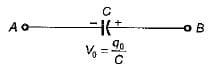Detailed Solution for Test: Capacitors & Inductors - 1 - Question 7

Due to initial condition, at t = 0 capacitor will act as a constant voltage source (at t = 0, capacitor acts as short-circuit). Hence, option (d) is correct.

Test: Capacitors & Inductors - 1 - Question 8

The strength of current in 1 Henry inductor changes at a rate of 1 A/sec. The magnitude of energy stored in the inductor after 3 sec is:

Detailed Solution for Test: Capacitors & Inductors - 1 - Question 8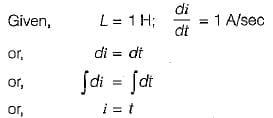∴ Current in the inductor after 3 sec is:

1= 3 A

Hence, energy is stored after 3 sec: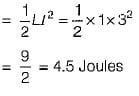Test: Capacitors & Inductors - 1 - Question 9

The current and voltage profile of an element vs time has been shown in given figure. The element and its value are respectively: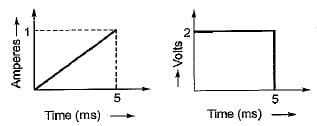Detailed Solution for Test: Capacitors & Inductors - 1 - Question 9
• Since V is not proportional to R therefore, the element can’t be a resistor.
• At t = 5 ms, even if i ≠ 0, the element behaves as a short circuit therefore, the element can’t be a capacitor (since at t = 0 only capacitor behaves as short circuit).
• The current at t = 0 is zero and at t = 5 ms voltage across the element is zero therefore, the element must be an inductor (at t = 0, an inductor acts as open circuit and at t =∞ it behaves as short circuit).
• From the given voltage and current profile, we have: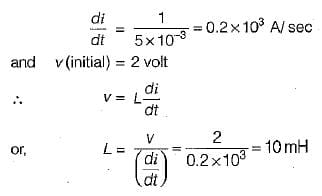Test: Capacitors & Inductors - 1 - Question 10

Figure shown below exhibits the voltage-time profile of a source to charge a capacitor of 50 μF. The value of charging current in amperes is: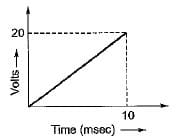Detailed Solution for Test: Capacitors & Inductors - 1 - Question 10

From given figure: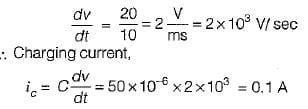Test: Capacitors & Inductors - 1 - Question 11

The equivalent capacitance across the given terminals A-B is: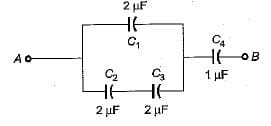Detailed Solution for Test: Capacitors & Inductors - 1 - Question 11
• The equivalent combination of C2 and C3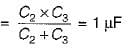• The equivalent combination of this 1 μF and  C1 = 2μF is C1+ 1 μF = 3μF
• Hence, the equivalent capacitance between terminals A and B is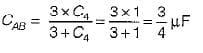Test: Capacitors & Inductors - 1 - Question 12

The charging time required to charge the equivalent capacitance between the given terminals a-b by a steady direct current of constant magnitude of 10 A is given by: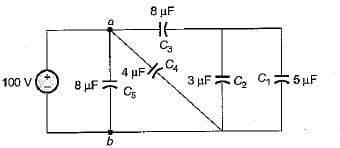Detailed Solution for Test: Capacitors & Inductors - 1 - Question 12

Equivalent capacitance between terminals a-b is: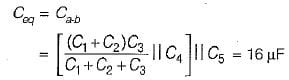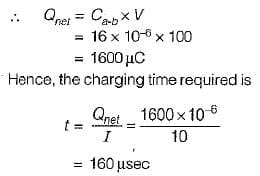Test: Capacitors & Inductors - 1 - Question 13

An ac voltage of 220 V is applied to a pure inductance of 50 H. If the current is 5 A, the instantaneous value of voltage and current will be respectively given by:

Detailed Solution for Test: Capacitors & Inductors - 1 - Question 13

Max. value of current: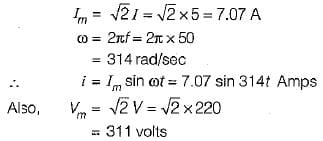Assuming voltage as the reference phasor: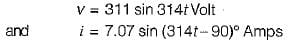Test: Capacitors & Inductors - 1 - Question 14

The voltage and current through a circuit element is v= 100 sin (314 t + 45°) volts and i = 10 sin (314 t - 45 ° ) amps.
The type of circuit element and its value will be respectively:

Detailed Solution for Test: Capacitors & Inductors - 1 - Question 14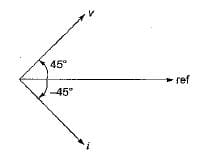The phase difference between v and i is: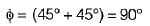Since v leads i therefore, the circuit element is an inductor.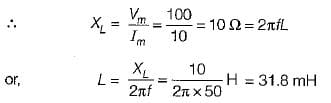Test: Capacitors & Inductors - 1 - Question 15

The equivalent inductance for the inductive circuit shown below at terminal “ 1 - 2 ” is: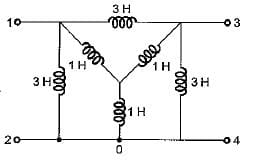Detailed Solution for Test: Capacitors & Inductors - 1 - Question 15

Converting the internal star connected inductance to an equivalent delta, the circuit reduces as shown below.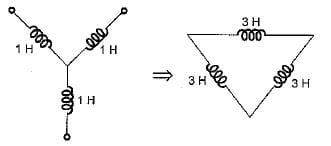Hence, equivalent circuit becomes as shown below.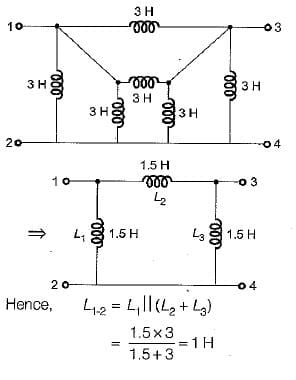## GATE Electrical Engineering (EE) 2023 Mock Test Series

22 docs|274 tests
Information about Test: Capacitors & Inductors - 1 Page
In this test you can find the Exam questions for Test: Capacitors & Inductors - 1 solved & explained in the simplest way possible. Besides giving Questions and answers for Test: Capacitors & Inductors - 1, EduRev gives you an ample number of Online tests for practice

## GATE Electrical Engineering (EE) 2023 Mock Test Series

22 docs|274 tests(Scan QR code)